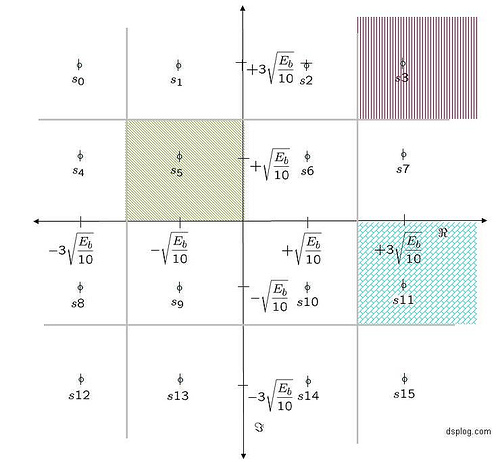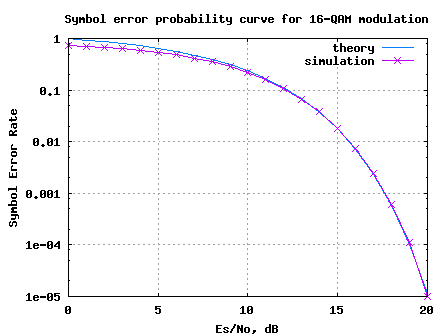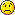(6 votes, average: 4.33 out of 5)Loading ...

# Symbol Error Rate (SER) for 16-QAM

by on December 9, 2007

Given that we have went over the symbol error probability for 4-PAM and symbol error rate for 4-QAM , let us extend the understanding to find the symbol error probability for 16-QAM (16 Quadrature Amplitude Modulation). Consider a typical 16-QAM modulation scheme where the alphabets (Refer example 5-37 in [DIG-COMM-BARRY-LEE-MESSERSCHMITT]).

are used.

The average energy of the 16-QAM constellation is (here). The 16-QAM constellation is as shown in the figure belowFigure: 16-QAM constellation

## Noise model

Assuming that the additive noise follows the Gaussian probability distribution function,

with and .

## Computing the probability of error

Consider the symbol in the inside, for example

The conditional probability distribution function (PDF) of given was transmitted is:

.

As can be seen from the above figure, the symbol is decoded correctly only if falls in the area in the black hashed region i.e.

.

Using the equations from (symbol error probability of 4-PAM as reference)

.

The probability of being decoded incorrectly is,

.

Consider the symbol in the corner, for example

The conditional probability distribution function (PDF) of given was transmitted is:

.

As can be seen from the above figure, the symbol is decoded correctly only if falls in the area in the red hashed region i.e.

.

Using the equations from (symbol error probability of 4-QAM as reference)

.

The probability of being decoded incorrectly is,

.

Consider the symbol which is not in the corner OR not in the inside, for example

The conditional probability distribution function (PDF) of given was transmitted is:

.

As can be seen from the above figure, the symbol is decoded correctly only if falls in the area in the blue hashed region i.e.

.

Using the above two cases are reference,

.

The probability of being decoded incorrectly is,

.

Total probability of symbol error

Assuming that all the symbols are equally likely (4 in the middle, 4 in the corner and the rest 8), the total probability of symbol error is,

.

## Simulation model

Simple Matlab/Octave code for generating 16QAM constellation, transmission through AWGN channel and computing the simulated symbol error rate.Figure: Symbol Error Rate curve for 16QAM modulation

Observations

1. Can observe that for low values, the theoretical results seem to be ‘pessimistic’ ‘optimistic’ compared to the simulated results. This is because for the approximated theoretical equation, the term was ignored. However, this approximation is valid only when the term is small, which need not be necessarily true for low values.

## Reference

[DIG-COMM-BARRY-LEE-MESSERSCHMITT]Digital Communication: Third Edition, by John R. Barry, Edward A. Lee, David G. MesserschmittHope this helps.

Krishna

D id you like this article? Make sure that you do not miss a new article by subscribing to RSS feed OR subscribing to e-mail newsletter. Note: Subscribing via e-mail entitles you to download the free e-Book on BER of BPSK/QPSK/16QAM/16PSK in AWGN.amin November 18, 2012 at 10:21 pm

In The name of God
This is the best educational website for me.
Thank you so much.Nadia July 28, 2012 at 1:38 pmNCH July 17, 2012 at 4:09 pm

hi
could you please send me 4QAM bit error rate code?
Thank youKrishna Sankar July 23, 2012 at 4:40 amVahid July 14, 2012 at 12:58 am

hello
please explain for me modulation 16qam by matlab codes
thanksKrishna Sankar July 14, 2012 at 6:21 am

@Vahid: This post does that. For bit error rate with Gray coded 16qam, please refer
http://www.dsplog.com/2008/06/05/16qam-bit-error-gray-mapping/ben hammouda imen March 13, 2012 at 8:00 pm

hi Krishna please i have a project on modelling simulation of OFDM on power line communication i need m-fileKrishna Sankar March 21, 2012 at 5:11 am

@ben: Sorry, I have not studied power line communicationhera May 6, 2011 at 11:28 am

hi… Im doing my project in 2D channel estimation for ofdm system. I have a doubt whether its possible to plot ( no of bits vs SNR plot) ., (SNR transitted vs SNR recieved).. please do help meKrishna Sankar May 24, 2011 at 5:33 am

@hera: Yes, plotting should not be a problemHassan November 17, 2010 at 6:24 am

Hi Krishna,
Thank you for your answerI have found it in your post

but I don’t know how implement for M-ary?
regards,Krishna Sankar November 18, 2010 at 4:56 am

@Hassan: Ah, good. In that code, by default, the simulation is for 64 QAM symol error rateHassan November 16, 2010 at 11:14 pm

Hi,
I need the Matlab code example for simulation of BER vs SNR for M-ary 64QAM.
Could you help me?
regards,
HassanKrishna Sankar November 17, 2010 at 4:38 am

Hassan: I have a post on 16 QAM Bit Error Rate @ http://www.dsplog.com/2008/06/05/16qam-bit-error-gray-mapping
Hope that helps.meps November 11, 2010 at 10:38 pm

Hello krishna, thank you for your articles, they are very helpful. Forgive me for bad english. I would like to ask why there is a deviation between the 2 curves in 16QAM in your two articles. I dont undrestand this, could you please help ?
I mean the above curve and the curve in this article http://www.eetimes.com/design/signal-processing-dsp/4017668/Modulation-roundup-error-rates-noise-and-capacity?pageNumber=0

ThanksKrishna Sankar November 14, 2010 at 10:29 am

@meps: What is the deviation which you are refering to? I was comparing Symbol Error Rate vs Es/N0 and both looked comparable.adi November 8, 2010 at 10:29 am

hi, i want to ask about 16 QAM matlab code under this web. why the mapping is a symbol for each bit? for N=256, your mapping result 256 symbol.
i read in some reference, 16 QAM has 1 symbol for every 4 bit. it means for N=256, will craete 64 symbol.
can you explain it? thanksKrishna Sankar November 15, 2010 at 1:51 am

@adi: For 16 QAM, each symbol carries 4 bit. The simulation code is computing Symbol error rate and not bit error rate.ASG October 19, 2010 at 3:23 am

How many phases and Amplitude does a 16-QAM have?Krishna Sankar November 17, 2010 at 5:31 am

@ASG: That one should be simple. Did you figure out the answer?abi June 21, 2010 at 1:33 pm

hi,
i need a code for 64 QAM modulation, also the BER and FER for this modulation, where the input is a sequence of ’0′ and ’1′.
thank youKrishna Sankar June 23, 2010 at 6:12 am

@abi: The matlab code in this post (for 16-QAM case) can be easily modified for the 64-QAM case.Guiyun LIU April 14, 2010 at 5:24 am

Wonderful Jobs.shadat December 6, 2009 at 2:45 pm

hi, plz could you send me 16QAM Bit Error Rate simulatio?Krishna Sankar December 7, 2009 at 5:29 amN.A October 3, 2009 at 7:05 pm

may i know the error probability (Pb) of 32-QAM..?? thks…Krishna Sankar October 8, 2009 at 5:20 amJules September 8, 2009 at 5:57 pm

hi!
I have simulated 4-QAM, 16QAM under AWGN and 4QAM under rayleigh fading and got good results. but when I simulate the 16QAM under rayleigh fading the decoder (MLD) hardly decodes even up to 50dB (BER=0.08102). Can anyone tell me what I’m doing wrong? because I’m only adding coefficient (y = h*x+n)
ThanksKrishna Sankar September 9, 2009 at 5:57 am

@Jules: Maybe your 16QAM demodulation is the culprit? You can have a look at 16QAM demodulator code @
http://www.dsplog.com/2007/12/09/symbol-error-rate-for-16-qam/
http://www.dsplog.com/2008/06/05/16qam-bit-error-gray-mapping/

Good luck.Jules October 5, 2009 at 3:45 pm

thank you Krishnabalu September 1, 2009 at 1:38 pm

hi…….
i am doing project in mimo signal detection using combined qrd-m and dfe detection technique for simple and efficient detection Can u please help me out with it ,thank u.Krishna Sankar September 7, 2009 at 5:36 am

@balu: You can email me your queries (but, sorry i cant do code debug). Good luck in your algorithm explorations.Altaf August 9, 2009 at 3:48 pm

Hi!
I am doing a project on digital hardware implementation of D64-QAM for voice band data transmission. Can u please help me out with it .Krishna Sankar August 11, 2009 at 4:42 am

@Altaf: Though I have not worked on the above mentioned technology, you can mail me with your queries. I will try answer to the best of my knowledge.soumendra August 3, 2009 at 7:46 pm

You have given the matlab code for simulating the SER of 16 QAM in AWGN channel. Can you provide some hints towards SER of 16 QAM in Rayleigh fading channel with diversity combining, i.e. how to incorporate the changes in your Matlab code given for AWGN case.Krishna Sankar August 5, 2009 at 5:53 am

a) First introduce only Rayleigh channel, have an equalizer as discussed in the post http://www.dsplog.com/2008/08/10/ber-bpsk-rayleigh-channel/ and obtain the reference curves
b) Once a) is done, introduce multiple receive chains and using the post http://www.dsplog.com/2008/09/28/maximal-ratio-combining/ as a reference, obtain the curves

Good luck.soumendra August 12, 2009 at 12:30 am

Thanks very much Krishna for your guidelines. I have still another query.

Regarding dual-hop Rayleigh channel (means between Tx and Rx, there exists another transceiver node), Can you provide some hints what would be the Equalization term if h1 and h2 are channel parameters in dual-hop case.Krishna Sankar August 14, 2009 at 4:59 am

@soumendra: Well, I would think that for each hop, you might be doing an equalization. Agree?soumendra August 17, 2009 at 5:34 pm

No, Sir. I am doing the equalization after two-hop by dividing the received signal by (h1.*h2). Which one is correct? after each hop or after two-hops.sarah July 25, 2009 at 3:39 am

hi
i wish i find any help in the topic of using ofdm in power line communication
if any one get any results by using matlab codes i wish i can have these results
thanksMelinda April 12, 2009 at 6:11 am

Hi,
Sorry for this new comment but just to be clear, I mean on figure with SER curvesMelinda April 12, 2009 at 6:07 am

Hi,

I think that on figure is an error(horizontal axes)
It is not realy Es/No but Eb/No .
(see MATLAB bertool for example)

Am I right?

Best regardsKrishna Sankar April 16, 2009 at 5:30 am

@Melinda: No, its indeed Es/N0. Was wondering, what made you think otherwise.Melinda March 21, 2009 at 5:55 am

Hi,

Why points on picture above are as Eb ?

On http://www.dsplog.com/2007/11/06/symbol-error-rate-for-4-qam/ – QPSK case is as Es?

Best regardsKrishna Sankar March 21, 2009 at 5:02 pm

@Melinda: Hmm… yes. I just checked. All the equations and BER plots are using Es/N0. However, I missed correcting the constellation plot diagram. Yes, the constellation point diagram should have shown Es/N0 (instead of Eb/N0).

Thanks for noticing and pointing that out. I will correct in future.Krishna Sankar October 31, 2008 at 6:06 am

@Philips: I do not know much about the channel model for power line communications (except that the channel is a wire, ofcourse).However, for OFDM aspect may I point to couple of articles which I have written in the past.
(a) Understanding an OFDM transmission
URI: http://www.dsplog.com/2008/02/03/understanding-an-ofdm-transmission/
(b) Cylcic prefix in Orthogonal Frequency Division Multiplexing
URI: http://www.dsplog.com/2008/02/17/cylcic-prefix-in-orthogonal-frequency-division-multiplexing/
(c) Peak to Average Power Ratio for OFDM
URI: http://www.dsplog.com/2008/02/24/peak-to-average-power-ratio-for-ofdm/Philips October 30, 2008 at 9:15 pm

Hi friends,
I’m studying a project which is a simulated OFDM on PLC(PowerLine Communication) with M-files in MatLab. I have not imaged my work yet. But I’ve read some documents which is described about OFDM and PLC(PowerLine Communication) models. Could you help me! Thank you so much.Tesla April 14, 2009 at 8:16 pm

me tooanybody help us

thanksKrishna Sankar April 17, 2009 at 5:51 am

@Tesla: I do not know much about the channel model for power line communications (except that the channel is a wire, ofcourse).However, for OFDM aspect may I point to couple of articles which I have written in the past.
(a) Understanding an OFDM transmission
URI: http://www.dsplog.com/2008/02/03/understanding-an-ofdm-transmission/
(b) Cylcic prefix in Orthogonal Frequency Division Multiplexing
URI: http://www.dsplog.com/2008/02/17/cylcic-prefix-in-orthogonal-frequency-division-multiplexing/
(c) Peak to Average Power Ratio for OFDM
URI: http://www.dsplog.com/2008/02/24/peak-to-average-power-ratio-for-ofdm/Krishna Sankar June 4, 2008 at 5:05 am

@hwan:
As you maybe aware, depending on the order of the modulation, constellation symbol might carry k bits, where k=log2(M). Typically k=1 for BPSK, 2 for QPSK, 4 for 16QAM etc.

The term SNR/bit refers to the energy required for transmitting a bit. So if the signal (constellation symbol) to noise ratio is Es/No, as each constellation carries k bits, then Es/No = k*Eb/No
Then term SNR/bit is equal to Eb/No.

Hope this helps.hwan June 3, 2008 at 3:07 pm

what is difference between Eb/No to SNR/bit.

many communication book show us case of SNR/bit.
i.e)John G.proakis ‘communication systems engineering’Krishna Sankar May 29, 2008 at 4:24 am

@rajesh: Well, for square QAM, you need to arrange them into {+/-1+/-j, +/-3,+/-3j,+/-3,+/-1j,+/-1,+/-3j} constellation points.rajesh May 27, 2008 at 7:07 pm

hi,
if i have 16 distinct points, what are the conditions on them to form 16 qam ???Krishna Sankar January 5, 2008 at 6:54 am

@mdkn45: Typically, we can assume that the bits assigned to constellation points are grey coded (i.e. bit groups allocated to adjacent symbols differ by only one bit). Which means that each symbol error causes only 1 out of 4 bits to be incorrectly detected. Given so, the bit error probability is 1/4th the symbol error probability.

Additionally, since we are transmitting 4 bits in a symbol,
the each symbol carries energy of four bits i.e Es/No = 4*Eb/No.

Combining, the bit error probability comes to
BER_{16QAM} = (3/8)*erfc(sqrt(4Eb/10No)).

Agree?mdkn45 January 4, 2008 at 11:36 am

hi, it’s very useful. But i want to bit error. What can i do?Krishna Sankar August 18, 2009 at 3:52 am

@soumendra: Well, I believe the idea behind using relay communication is to improve the reliability of the link. So, the intermediete node might be doing something. Couple of ways are:
a) decode the data, and then re-transmit.
b) amplify the signal + noise, and then re-transmit.
c) more ?

Depending on your system design, you may chose the appropriate approach.soumendra August 18, 2009 at 4:34 pm

Yes, Sir…. I have adopted the strategy (b) amplify the signal + noise, and then re-transmit….So, the equalization term after two-hop would be dividing the received signal by (G*h1.*h2) where G is the amplification factor. Am I right?Krishna Sankar August 19, 2009 at 5:37 am

@soumendra: Yes, it sounds correct.
y1 = h1x + n1
y2 = h2*g*y1+n2 = h2*g*(h1x+n1) + n2 = h2gh1x + h2n1 + n2soumendra August 20, 2009 at 3:50 pm

Other way could be doing equalization after each hop, i.e.
y1 = h1x + n1; y1=y1/h1;

y2 = h2*g*y1+n2 = h2*g*(x+(n1/h1)) + n2 = h2*g*x + g*(h2/h1)*n1 + n2;

y2=y2/(g*h2);

Which one would be appropriate ? doing equalization after each hop or after two-hop.Krishna Sankar August 22, 2009 at 5:11 am